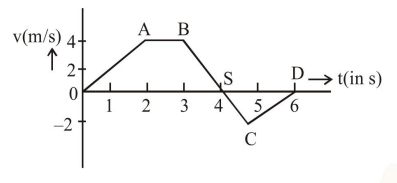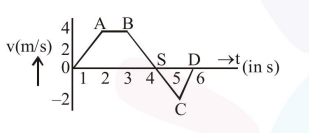# The velocity (v) and time (t) graph of a body in a straight line

Question:

The velocity (v) and time (t) graph of a body in a straight line motion is shown in the figure. The point $S$ is at $4.333$ seconds. The total distance covered by the body in $6 \mathrm{~s}$ is :1. $12 \mathrm{~m}$

2. $\frac{49}{4} \mathrm{~m}$

3. $11 \mathrm{~m}$

4. $\frac{37}{3} \mathrm{~m}$

Correct Option: , 4

Solution:$\mathrm{OS}=4+\frac{1}{3}=\frac{13}{3}$

$\mathrm{SD}=2-\frac{1}{3}=\frac{5}{3}$

Area of $\mathrm{OABS}$ is $\mathrm{A}_{1}$

Area of $S C D$ is $A_{2}$

Distance $=\left|\mathrm{A}_{1}\right|+\left|\mathrm{A}_{2}\right|$

$\mathrm{A}_{1}=\frac{1}{2}\left[\frac{13}{3}+1\right] 4=\frac{32}{3}$

Distance $=\left|\mathrm{A}_{1}\right|+\left|\mathrm{A}_{2}\right|$

$=\frac{32}{3}+\frac{5}{3}$

$=\frac{37}{3}$